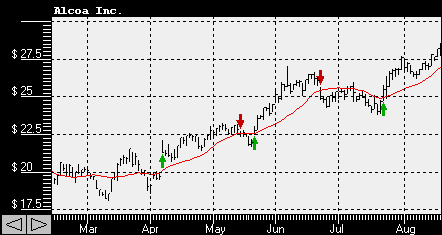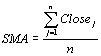# Moving Averages - Technical Indicators

Moving averages is one of the most popular technical indicators. Moving averages show and average value of the stock price over the period of time. Moving averages are often compared to the security price itself or to the moving averages of securities price on a different time span.Typically a buy signal is generated when a securities price rises above moving average and a sell signal is generated when the securities price falls below moving average. There are several types of moving averages: simple moving average, exponential moving average, triangular moving average, variable and weighted moving average. Moving averages can be calculated on any data series and often are used as a part of other technical indicators. Commonly used simple moving average is calculated as following:where n is the number of days and Close(j) is the closing stock price on a day j.